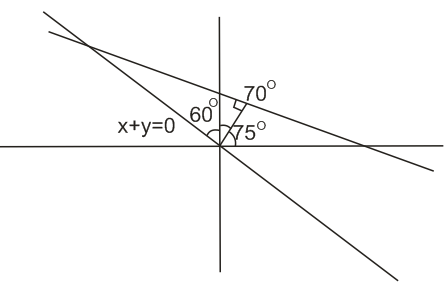Q

# Help me understand! - Co-ordinate geometry - JEE Main-7

The straight line L at a distance of 4 units from the origin makes

positive intercepts on the coordinate axes and the perpendicular

from the origin to this line makes an angle of  with the line

. Then an equation of the line L is :

• Option 1)

• Option 2)

• Option 3)

• Option 4)

ViewsHence, the equation of line is

Option 1)

Option 2)

Option 3)

Option 4)

Exams
Articles
Questions